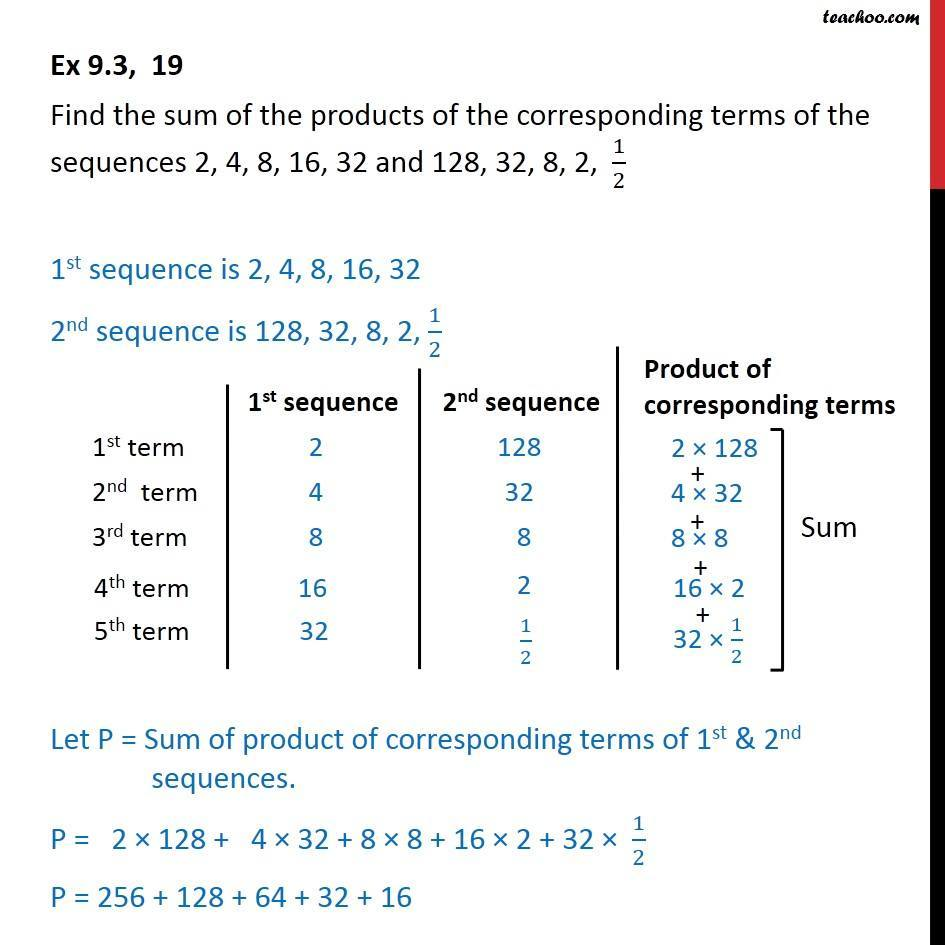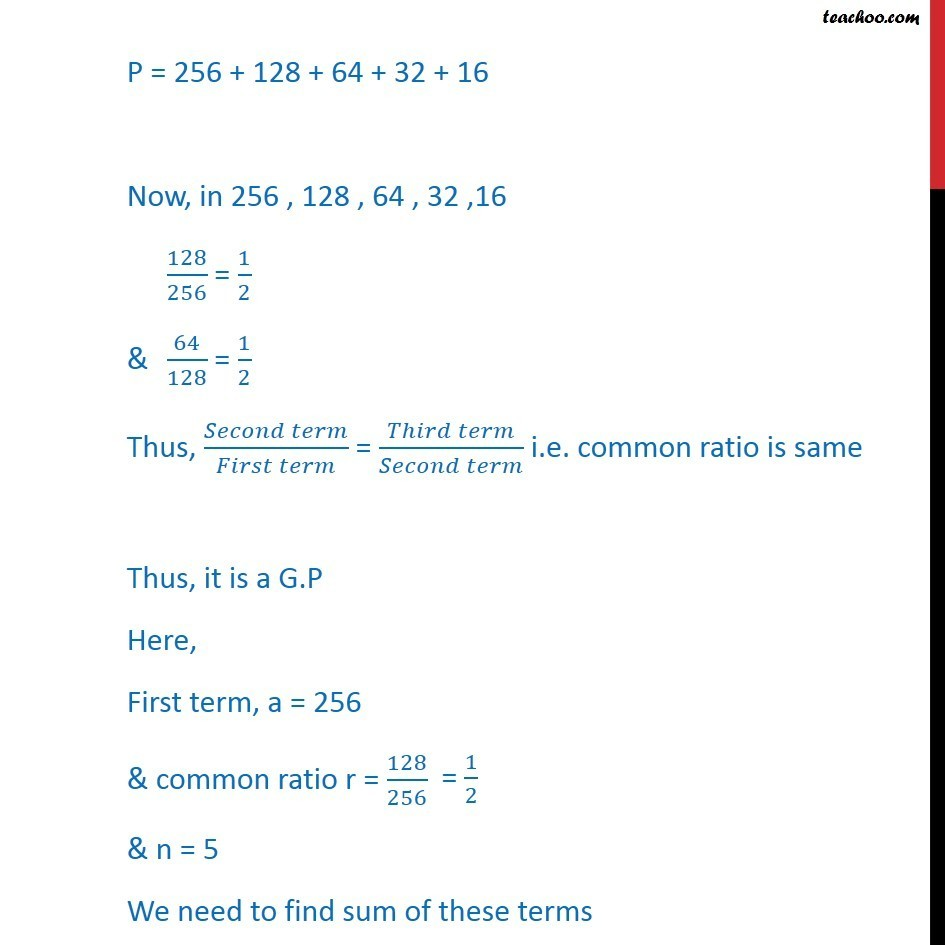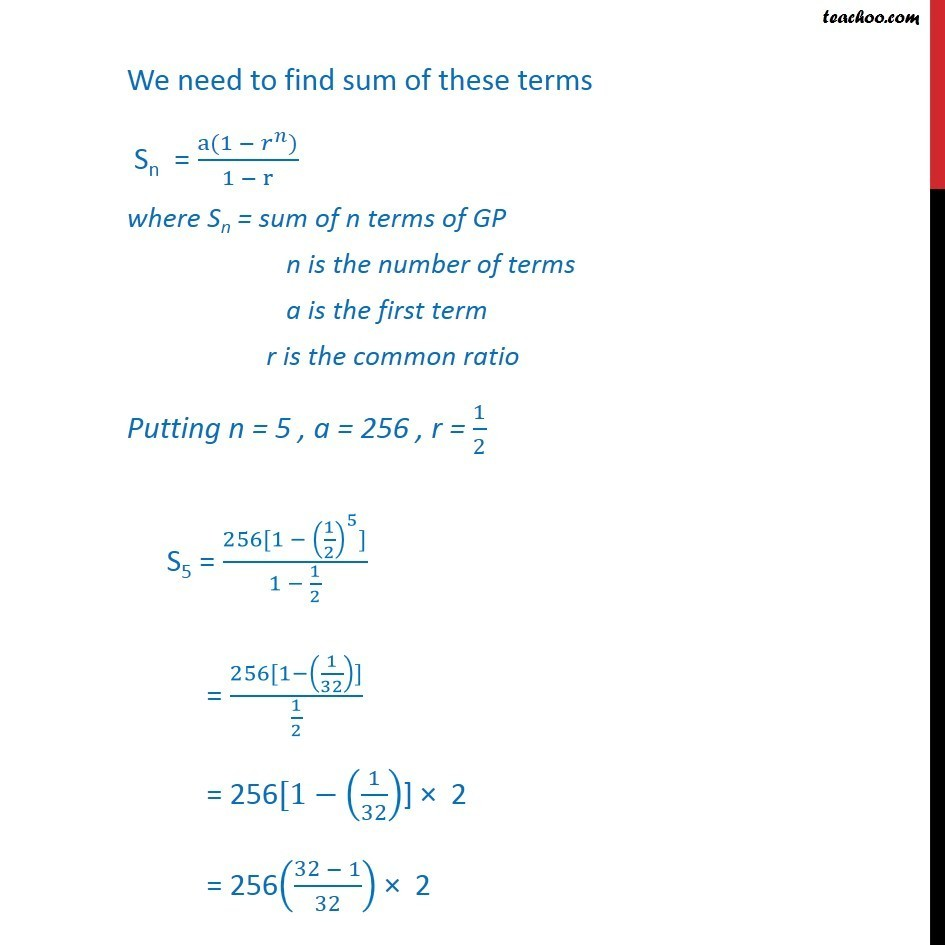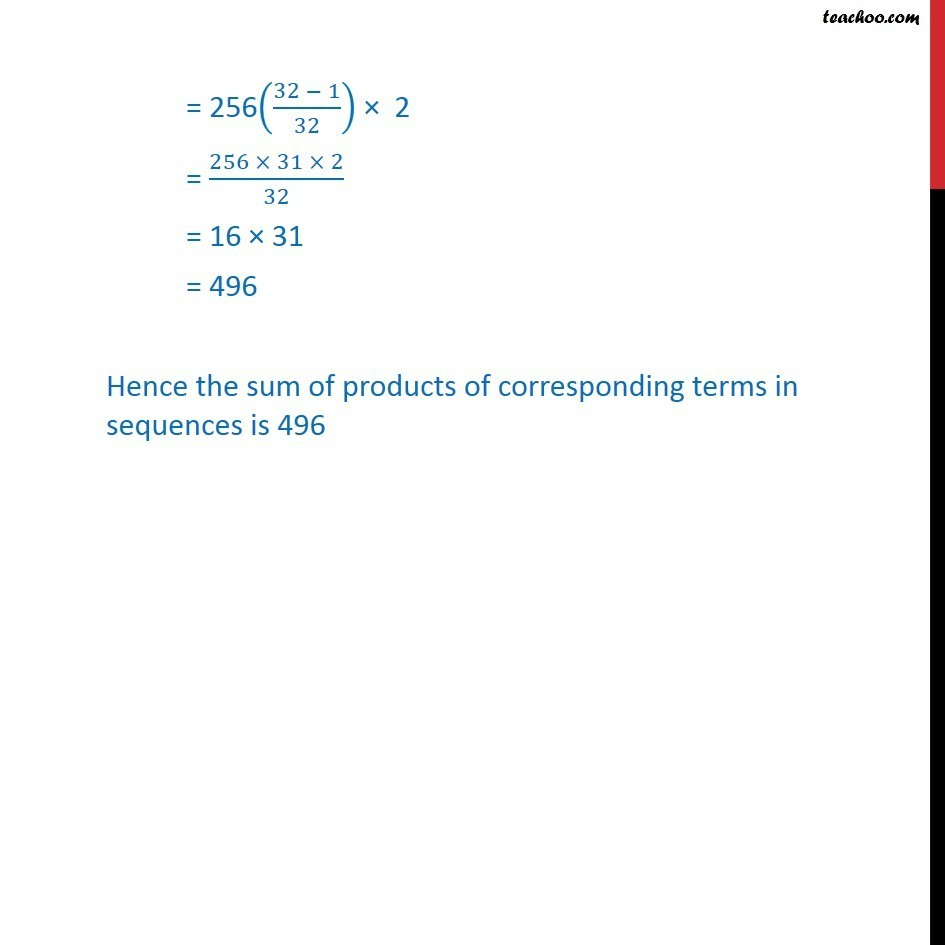Geometric Progression(GP): Formulae based

Chapter 8 Class 11 Sequences and Series
Concept wiseLearn in your speed, with individual attention - Teachoo Maths 1-on-1 Class

### Transcript

Ex 8.2, 19 Find the sum of the products of the corresponding terms of the sequences 2, 4, 8, 16, 32 and 128, 32, 8, 2, 1/2 1st sequence is 2, 4, 8, 16, 32 2nd sequence is 128, 32, 8, 2, 1/2 Let P = Sum of product of corresponding terms of 1st & 2nd sequences. P = 2 128 + 4 32 + 8 8 + 16 2 + 32 1/2 P = 256 + 128 + 64 + 32 + 16 P = 256 + 128 + 64 + 32 + 16 Now, in 256 , 128 , 64 , 32 ,16 128/256 = 1/2 & 64/128 = 1/2 Thus, ( )/( ) = ( )/( ) i.e. common ratio is same Thus, it is a G.P Here, First term, a = 256 & common ratio r = 128/256 & n = 5 We need to find sum of these terms We need to find sum of these terms Sn = (a(1 ^ ))/(1 r) where Sn = sum of n terms of GP n is the number of terms a is the first term r is the common ratio Putting n = 5 , a = 256 , r = 1/2 S5 = (256[1 (1/2)^5])/(1 1/2) = (256[1 (1/32)])/(1/2) = 256[1 (1/32)] 2 = 256((32 1)/32) 2 = 256((32 1)/32) 2 = (256 31 2)/32 = 16 31 = 496 Hence the sum of products of corresponding terms in sequences is 496